7-2 GMM Application: PDF Modeling

[chinese][all]

In the previous section, we have covered the mathematics of EM (expectation maximization) which, under the framework of MLE, can be employed to identify the optimum parameters for a GMM. In this section, we shall demonstrate the use of GMM for PDF modeling.

For the first example, we shall use GMM for modeling the probability density function of a 1D data, as follows.

Example 1: gmm1d01.m% GMM (gaussian mixture model) for 1-D data % ====== Plot data histogram DS = dcData(8); data = DS.input; subplot(2,1,1); binNum = 30; hist(data, binNum); xlabel('Data values'); ylabel('Counts'); title('Data histogram'); colormap(summer); % ====== Train GMM gmmOpt=gmmTrain('defaultOpt'); gmmOpt.arch.covType=1; gmmOpt.arch.gaussianNum = 3; [gmmPrm, logLike]=gmmTrain(data, gmmOpt); % ====== Plot the log likelihood subplot(2,1,2); plot(logLike, 'o-'); xlabel('No. of iterations of GMM training'); ylabel('Log likelihood'); % ====== Print the result fprintf('w1=%g, mu1=%g, sigma1=%g\n', gmmPrm(1).w, gmmPrm(1).mu, gmmPrm(1).sigma); fprintf('w2=%g, mu2=%g, sigma2=%g\n', gmmPrm(2).w, gmmPrm(2).mu, gmmPrm(2).sigma); fprintf('w3=%g, mu3=%g, sigma3=%g\n', gmmPrm(3).w, gmmPrm(3).mu, gmmPrm(3).sigma); fprintf('Overall logLike = %g\n', sum(log(gmmEval(data, gmmPrm))));w1=0.476411, mu1=2.01478, sigma1=0.240201 w2=0.165196, mu2=-1.99372, sigma2=0.105661 w3=0.358393, mu3=0.122917, sigma3=1.15757 Overall logLike = 2568.89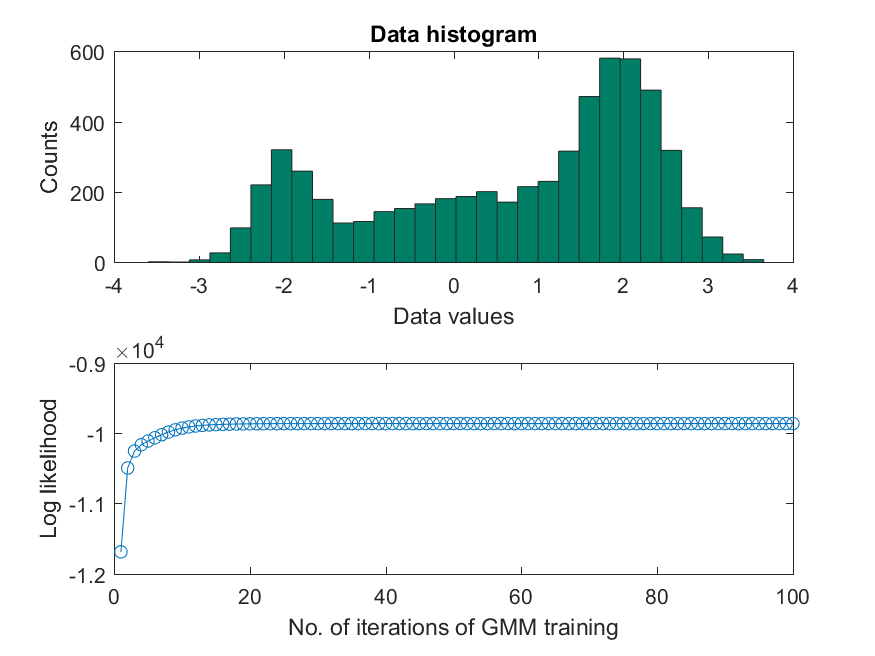In the previous example, the data is generated via three Gaussian PDFs centered at -2, 0, 2 (please refer to the contents of dcData.m). The first plot is the histogram of the dataset; the second plot is the curve of log probability w.r.t. the number of iterations. From the above example, we have the following observations:

1. The identified centers are very close to the means of the three Gaussian PDF.
2. Log probability is monotonically nondecreasing throughout the training iterations.

We can use the following example to plot the PDF after training:

Example 2: gmm1d02.m% GMM (gaussian mixture model) for 1-D data % ====== Plot the histogram clf DS = dcData(8); data = DS.input; subplot(2,1,1); binNum = 30; hist(data, binNum); xlabel('Data values'); ylabel('Counts'); title('Data histogram'); colormap(summer); % ====== Perform GMM training gmmOpt=gmmTrain('defaultOpt'); gmmOpt.arch.gaussianNum = 3; [gmmPrm, logLike]=gmmTrain(data, gmmOpt); % ====== Plot the PDF x = linspace(min(data), max(data)); subplot(2,1,2); hold on for i = 1:gmmOpt.arch.gaussianNum, h1 = plot(x, gaussian(x, gmmPrm(i)), '--m'); set(h1, 'linewidth', 2); end for i = 1:gmmOpt.arch.gaussianNum, h2 = plot(x, gaussian(x, gmmPrm(i))*gmmPrm(i).w, ':b'); set(h2, 'linewidth', 2); end total = zeros(size(x)); for i = 1:gmmOpt.arch.gaussianNum, g(i,:)=gaussian(x, gmmPrm(i)); total=total+g(i, :)*gmmPrm(i).w; end h3 = plot(x, total, 'r'); set(h3, 'linewidth', 2); hold off box on legend([h1 h2 h3], 'g_i', 'w_ig_i', '\Sigma_i w_ig_i'); xlabel('Data values'); ylabel('Prob.'); title('Gaussian mixture model'); % Print texts of g1, g2, g3 for i=1:gmmOpt.arch.gaussianNum [maxValue, index]=max(g(i, :)); text(x(index), maxValue, ['g_', int2str(i)], 'vertical', 'bottom', 'horizon', 'center'); end % ====== Plot the curve on top of the histogram k = size(data,2)*(max(data)-min(data))/binNum; subplot(2,1,1) line(x, total*k, 'color', 'r', 'linewidth', 2); % Plot the data axisLimit=axis; line(data, rand(length(data),1)*(axisLimit(4)-axisLimit(3))/10+axisLimit(3), 'marker', '.', 'linestyle', 'none', 'color', 'k');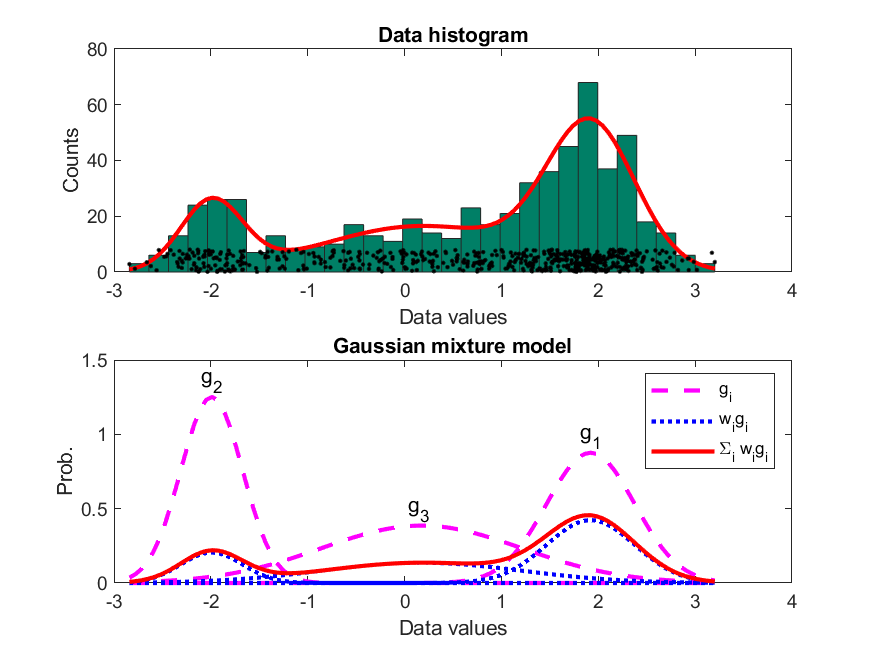From the above example, the identified GMM PDF can match the data histogram closely. This is based on the following three conditions:

1. The size of data is large enough. (The above example has 600 data entries.)
2. We are able to guess the number of Gaussians correctly.
3. The data is indeed governed by GMM.

In practice, the above three conditions do not always hold. The basic remedies are:

1. Try to collect as much data as possible.
2. Use some heuristic search to find the optimum number of Gaussian PDFs.
3. Increase the number of mixtures so we can approximate any PDF using the training data.

In the following example, we should use GMM to model the 2D donut dataset, as follows:

Example 3: gmm2d01.m% GMM (gaussian mixture model) for 2-D "donut" data % ====== Get data and train GMM DS = dcData(2); data=DS.input; gmmOpt=gmmTrain('defaultOpt'); gmmOpt.arch.covType=1; gmmOpt.arch.gaussianNum=6; gmmOpt.train.showInfo=1; gmmOpt.train.useKmeans=0; gmmOpt.train.maxIteration=50; [gmmPrm, logLike] = gmmTrain(data, gmmOpt); % ====== Plot log likelihood figure; subplot(2,2,1) plot(logLike, 'o-'); xlabel('No. of iterations of GMM training'); ylabel('Log likelihood'); % ====== Plot scattered data and positions of the Gaussians subplot(2,2,2); plot(data(1,:), data(2,:),'.r'); axis image theta=linspace(-pi, pi, 21); circleH=zeros(1, length(gmmPrm)); for i=1:length(gmmPrm) r=sqrt(2*log(2)*gmmPrm(i).sigma); % Gaussian reaches it's 50% height at this distance from the mean xData=r*cos(theta)+gmmPrm(i).mu(1); yData=r*sin(theta)+gmmPrm(i).mu(2); circleH(i)=line(xData, yData, 'color', 'k', 'linewidth', 3); end % ====== Surface/contour plots pointNum = 40; x = linspace(min(data(1,:)), max(data(1,:)), pointNum); y = linspace(min(data(2,:)), max(data(2,:)), pointNum); [xx, yy] = meshgrid(x, y); data = [xx(:) yy(:)]'; z = gmmEval(data, gmmPrm); zz = reshape(z, pointNum, pointNum); subplot(2,2,3); mesh(xx, yy, zz); axis tight; box on; rotate3d on subplot(2,2,4); contour(xx, yy, zz, 30); axis image GMM iteration: 0/50, log likelihood. = -2459.111841 GMM iteration: 1/50, log likelihood. = -1889.343285 GMM iteration: 2/50, log likelihood. = -1820.970204 GMM iteration: 3/50, log likelihood. = -1747.493220 GMM iteration: 4/50, log likelihood. = -1676.794974 GMM iteration: 5/50, log likelihood. = -1626.868731 GMM iteration: 6/50, log likelihood. = -1602.955088 GMM iteration: 7/50, log likelihood. = -1593.969408 GMM iteration: 8/50, log likelihood. = -1590.077032 GMM iteration: 9/50, log likelihood. = -1587.646618 GMM iteration: 10/50, log likelihood. = -1585.656179 GMM iteration: 11/50, log likelihood. = -1583.849816 GMM iteration: 12/50, log likelihood. = -1582.163698 GMM iteration: 13/50, log likelihood. = -1580.580310 GMM iteration: 14/50, log likelihood. = -1579.094987 GMM iteration: 15/50, log likelihood. = -1577.707271 GMM iteration: 16/50, log likelihood. = -1576.417712 GMM iteration: 17/50, log likelihood. = -1575.226285 GMM iteration: 18/50, log likelihood. = -1574.131712 GMM iteration: 19/50, log likelihood. = -1573.131390 GMM iteration: 20/50, log likelihood. = -1572.221714 GMM iteration: 21/50, log likelihood. = -1571.398523 GMM iteration: 22/50, log likelihood. = -1570.657468 GMM iteration: 23/50, log likelihood. = -1569.994208 GMM iteration: 24/50, log likelihood. = -1569.404414 GMM iteration: 25/50, log likelihood. = -1568.883662 GMM iteration: 26/50, log likelihood. = -1568.427301 GMM iteration: 27/50, log likelihood. = -1568.030370 GMM iteration: 28/50, log likelihood. = -1567.687602 GMM iteration: 29/50, log likelihood. = -1567.393525 GMM iteration: 30/50, log likelihood. = -1567.142610 GMM iteration: 31/50, log likelihood. = -1566.929448 GMM iteration: 32/50, log likelihood. = -1566.748905 GMM iteration: 33/50, log likelihood. = -1566.596242 GMM iteration: 34/50, log likelihood. = -1566.467191 GMM iteration: 35/50, log likelihood. = -1566.357986 GMM iteration: 36/50, log likelihood. = -1566.265359 GMM iteration: 37/50, log likelihood. = -1566.186514 GMM iteration: 38/50, log likelihood. = -1566.119085 GMM iteration: 39/50, log likelihood. = -1566.061082 GMM iteration: 40/50, log likelihood. = -1566.010847 GMM iteration: 41/50, log likelihood. = -1565.966998 GMM iteration: 42/50, log likelihood. = -1565.928391 GMM iteration: 43/50, log likelihood. = -1565.894077 GMM iteration: 44/50, log likelihood. = -1565.863269 GMM iteration: 45/50, log likelihood. = -1565.835317 GMM iteration: 46/50, log likelihood. = -1565.809678 GMM iteration: 47/50, log likelihood. = -1565.785900 GMM iteration: 48/50, log likelihood. = -1565.763607 GMM iteration: 49/50, log likelihood. = -1565.742482 GMM total iteration count = 50, log likelihood. = -1565.722259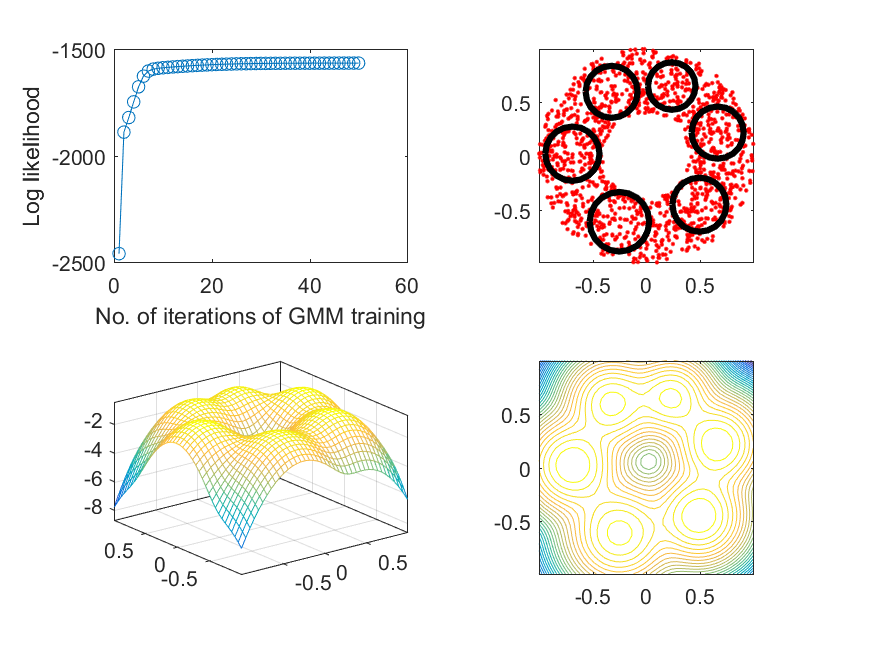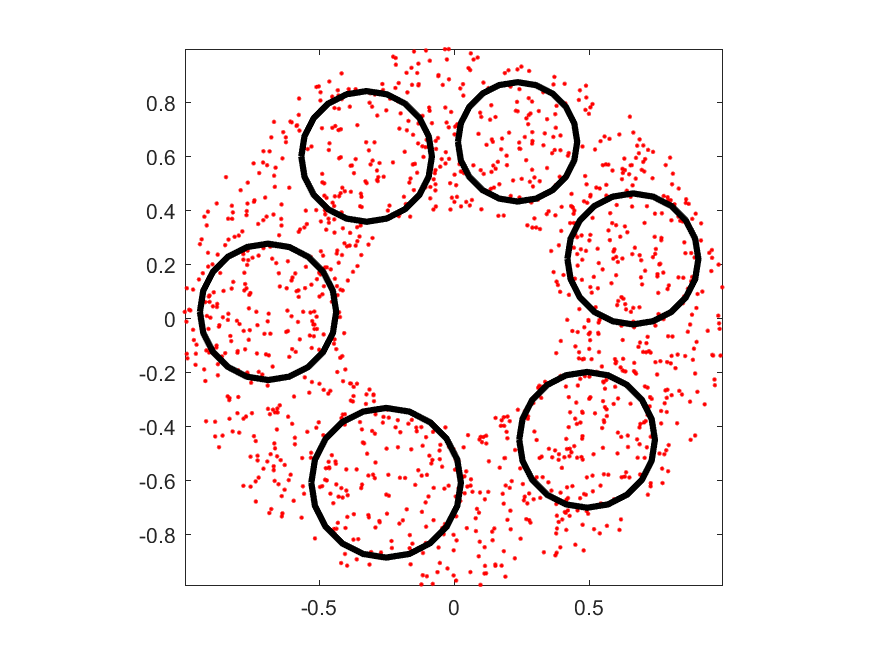In the above example, you should be able to see the flashy animation during the training process. Moreover, since we have set gmmPrm.useKmeans=0, the training process will randomly select several data points as the initial centers instead of using k-means for determining a set of better centers. Since the initial centers are randomly selected, the program will need more time to adjust these 6 Gaussians.

Not every dataset modeled by GMM will generate satisfactory result. An example follows.

Example 4: gmm2d02.m% GMM (gaussian mixture model) for 2-D "uneven" data % ====== Get data and train GMM DS = dcData(4); data=DS.input; gmmOpt=gmmTrain('defaultOpt'); gmmOpt.arch.covType=1; gmmOpt.arch.gaussianNum=3; gmmOpt.train.showInfo=1; gmmOpt.train.useKmeans=0; gmmOpt.train.maxIteration=30; close all; [gmmPrm, logLike] = gmmTrain(data, gmmOpt); % ====== Plot log prob. figure; subplot(2,2,1) plot(logLike, 'o-'); xlabel('No. of iterations of GMM training'); ylabel('Log likelihood'); % ====== Plot scattered data and positions of the Gaussians subplot(2,2,2); plot(data(1,:), data(2,:),'.r'); axis image theta=linspace(-pi, pi, 21); for i=1:length(gmmPrm) r=sqrt(2*log(2)*gmmPrm(i).sigma); % Gaussian reaches it's 50% height at this distance from the mean xData=r*cos(theta)+gmmPrm(i).mu(1); yData=r*sin(theta)+gmmPrm(i).mu(2); circleH(i)=line(xData, yData, 'color', 'k', 'linewidth', 3); end % ====== Surface/contour plots pointNum = 40; x = linspace(min(data(1,:)), max(data(1,:)), pointNum); y = linspace(min(data(2,:)), max(data(2,:)), pointNum); [xx, yy] = meshgrid(x, y); data = [xx(:) yy(:)]'; z = gmmEval(data, gmmPrm); zz = reshape(z, pointNum, pointNum); subplot(2,2,3); mesh(xx, yy, zz); axis tight; box on; rotate3d on subplot(2,2,4); contour(xx, yy, zz, 30); axis image GMM iteration: 0/30, log likelihood. = -8241.699335 GMM iteration: 1/30, log likelihood. = -8014.746943 GMM iteration: 2/30, log likelihood. = -7972.337933 GMM iteration: 3/30, log likelihood. = -7938.832854 GMM iteration: 4/30, log likelihood. = -7871.371995 GMM iteration: 5/30, log likelihood. = -7701.595858 GMM iteration: 6/30, log likelihood. = -7471.854821 GMM iteration: 7/30, log likelihood. = -7333.726813 GMM iteration: 8/30, log likelihood. = -7259.232890 GMM iteration: 9/30, log likelihood. = -7240.661757 GMM iteration: 10/30, log likelihood. = -7239.320100 GMM iteration: 11/30, log likelihood. = -7239.221211 GMM iteration: 12/30, log likelihood. = -7239.213669 GMM iteration: 13/30, log likelihood. = -7239.213078 GMM iteration: 14/30, log likelihood. = -7239.213031 GMM iteration: 15/30, log likelihood. = -7239.213028 GMM iteration: 16/30, log likelihood. = -7239.213027 GMM iteration: 17/30, log likelihood. = -7239.213027 GMM iteration: 18/30, log likelihood. = -7239.213027 GMM iteration: 19/30, log likelihood. = -7239.213027 GMM iteration: 20/30, log likelihood. = -7239.213027 GMM iteration: 21/30, log likelihood. = -7239.213027 GMM iteration: 22/30, log likelihood. = -7239.213027 GMM total iteration count = 23, log likelihood. = -7239.213027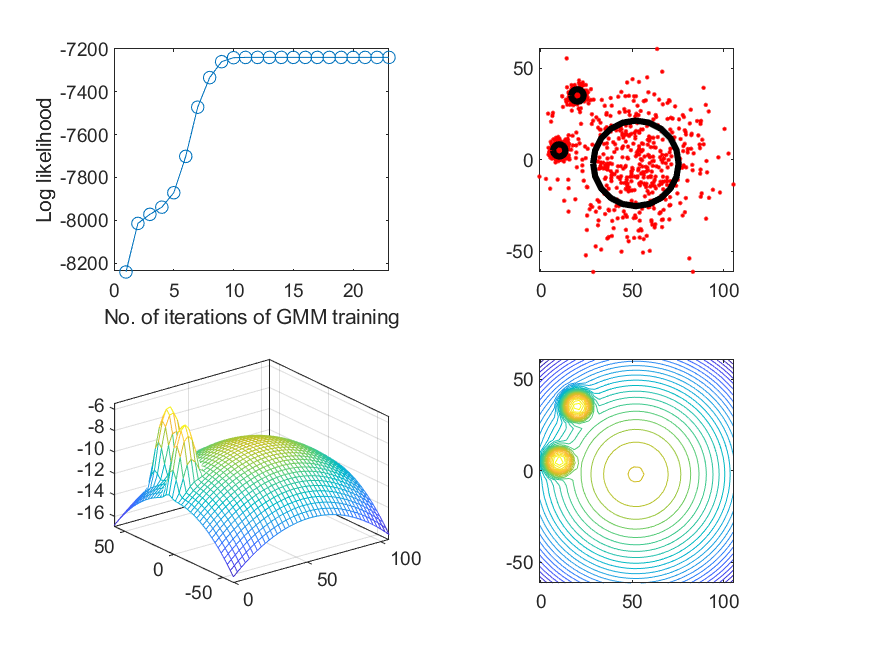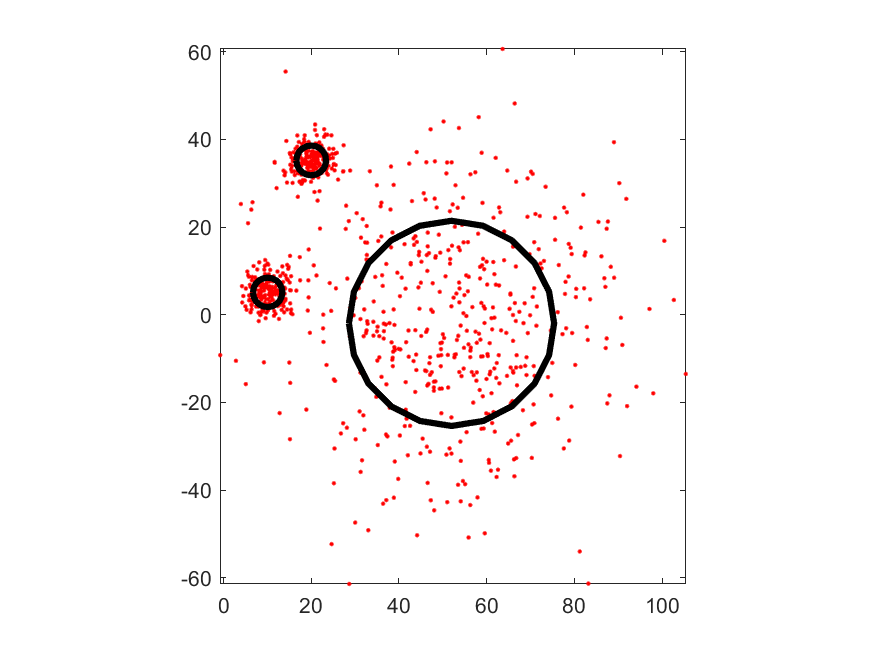Judging from the scatter plot of the data set, we should have three Gaussians to cover the three clusters. The first two at the upper left corner should be sharper while the third one at the center should be flatter. In practice, it is likely to have the situation with "big circle surrounds small one", indicating the training process was trapped in a local maximum. (Since the data is randomly generated, you should try the program several times to obtain several possible results.)

Here is another example of GMM modeling (using 4 Gaussians) over 2D data:

Example 5: gmm2d03.m% GMM (gaussian mixture model) for 2-D "donut" data % ====== Get data and train GMM DS = dcData(6); data=DS.input; gmmOpt=gmmTrain('defaultOpt'); gmmOpt.arch.covType=3; gmmOpt.arch.gaussianNum=4; gmmOpt.train.showInfo=1; gmmOpt.train.useKmeans=1; gmmOpt.train.maxIteration=50; [gmmPrm, logLike] = gmmTrain(data, gmmOpt, 1); Start KMEANS to find the initial mean vectors... GMM iteration: 0/50, log likelihood. = -226.515201 GMM iteration: 1/50, log likelihood. = -110.237816 GMM iteration: 2/50, log likelihood. = -94.557989 GMM iteration: 3/50, log likelihood. = -67.502974 GMM iteration: 4/50, log likelihood. = -33.783473 GMM iteration: 5/50, log likelihood. = -1.456188 GMM iteration: 6/50, log likelihood. = 22.854160 GMM iteration: 7/50, log likelihood. = 32.707306 GMM iteration: 8/50, log likelihood. = 35.758555 GMM iteration: 9/50, log likelihood. = 36.725392 GMM iteration: 10/50, log likelihood. = 37.110098 GMM iteration: 11/50, log likelihood. = 37.299703 GMM iteration: 12/50, log likelihood. = 37.400726 GMM iteration: 13/50, log likelihood. = 37.455973 GMM iteration: 14/50, log likelihood. = 37.486577 GMM iteration: 15/50, log likelihood. = 37.503679 GMM iteration: 16/50, log likelihood. = 37.513298 GMM iteration: 17/50, log likelihood. = 37.518736 GMM iteration: 18/50, log likelihood. = 37.521823 GMM iteration: 19/50, log likelihood. = 37.523580 GMM iteration: 20/50, log likelihood. = 37.524583 GMM iteration: 21/50, log likelihood. = 37.525156 GMM iteration: 22/50, log likelihood. = 37.525485 GMM iteration: 23/50, log likelihood. = 37.525673 GMM iteration: 24/50, log likelihood. = 37.525781 GMM iteration: 25/50, log likelihood. = 37.525843 GMM iteration: 26/50, log likelihood. = 37.525879 GMM iteration: 27/50, log likelihood. = 37.525899 GMM iteration: 28/50, log likelihood. = 37.525911 GMM iteration: 29/50, log likelihood. = 37.525918 GMM iteration: 30/50, log likelihood. = 37.525921 GMM iteration: 31/50, log likelihood. = 37.525924 GMM iteration: 32/50, log likelihood. = 37.525925 GMM iteration: 33/50, log likelihood. = 37.525926 GMM iteration: 34/50, log likelihood. = 37.525926 GMM iteration: 35/50, log likelihood. = 37.525926 GMM iteration: 36/50, log likelihood. = 37.525927 GMM iteration: 37/50, log likelihood. = 37.525927 GMM iteration: 38/50, log likelihood. = 37.525927 GMM iteration: 39/50, log likelihood. = 37.525927 GMM iteration: 40/50, log likelihood. = 37.525927 GMM iteration: 41/50, log likelihood. = 37.525927 GMM iteration: 42/50, log likelihood. = 37.525927 GMM iteration: 43/50, log likelihood. = 37.525927 GMM iteration: 44/50, log likelihood. = 37.525927 GMM iteration: 45/50, log likelihood. = 37.525927 GMM iteration: 46/50, log likelihood. = 37.525927 GMM iteration: 47/50, log likelihood. = 37.525927 GMM iteration: 48/50, log likelihood. = 37.525927 GMM iteration: 49/50, log likelihood. = 37.525927 GMM total iteration count = 50, log likelihood. = 37.525927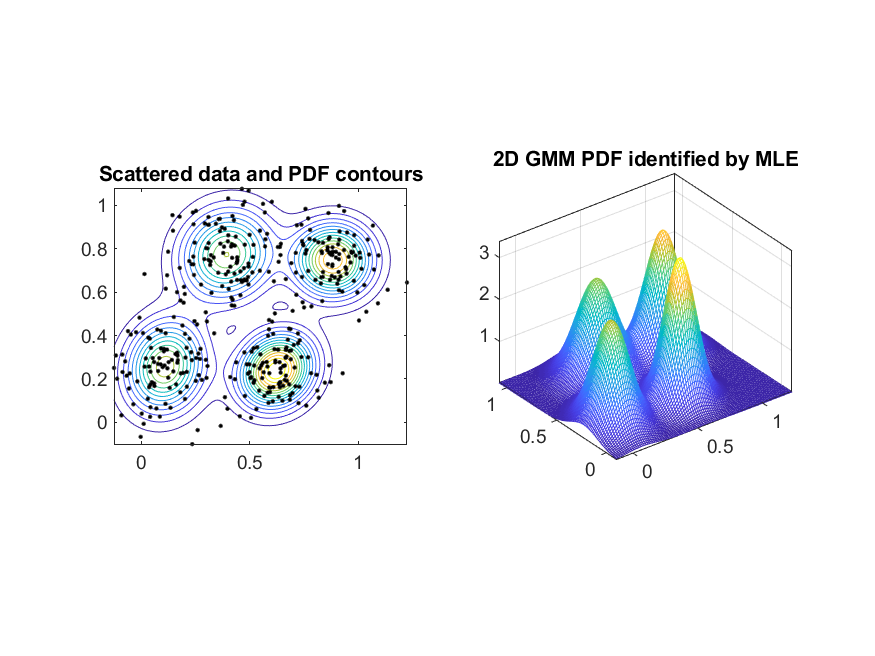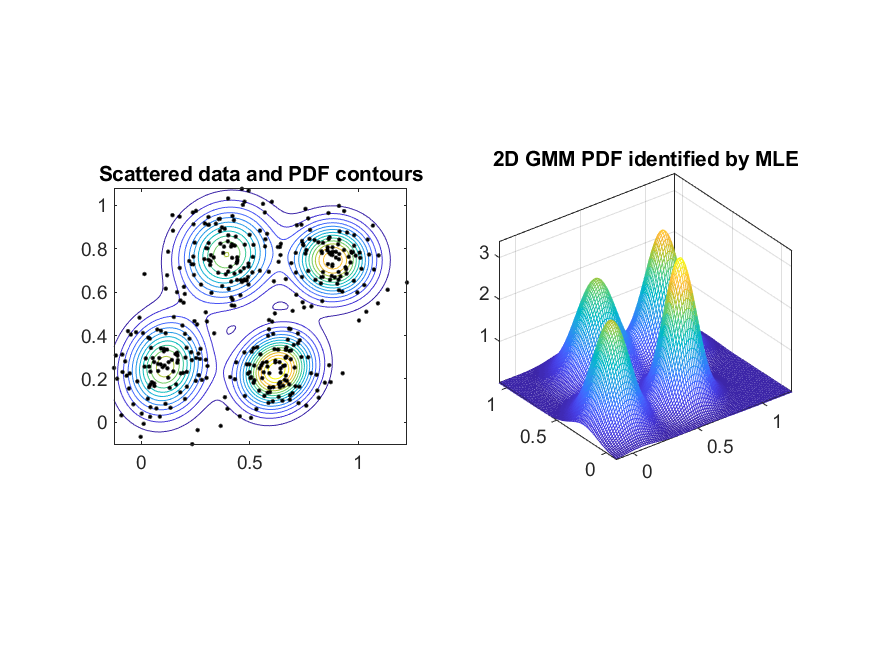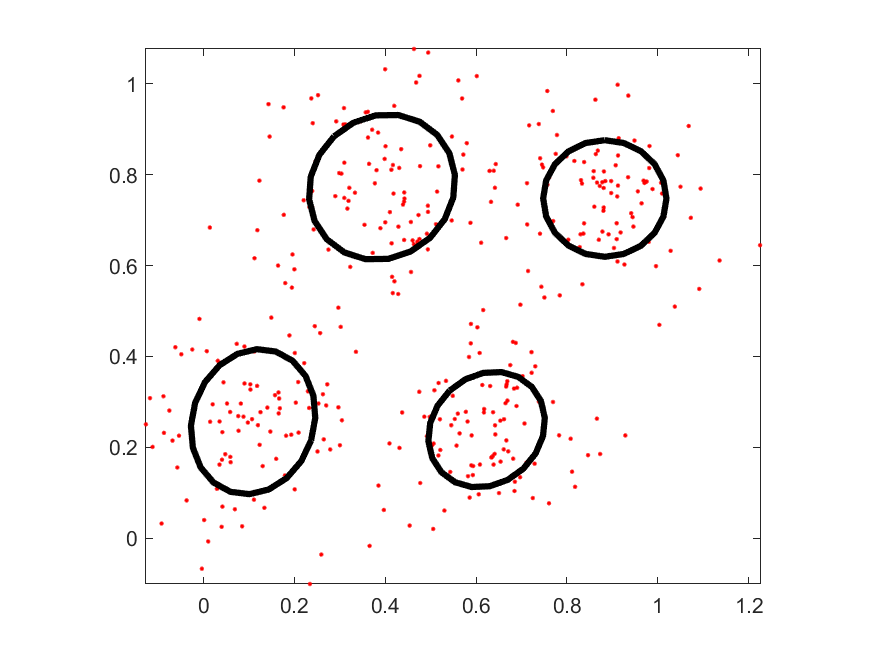Data Clustering and Pattern Recognition (資料分群與樣式辨認)